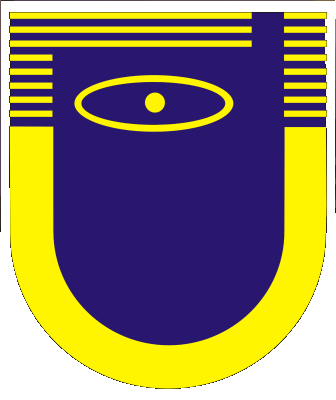Main Page
Subscription information

Current Issue
Tables of Contents
Author Index
Search

Authors
Referees

## Unsteady flow of real conducting gas in a MHD channel with continuous electrodes

A. I. Bertinov - D. A. But - L. K. Kovalev - S. M. Koneev

Abstract
The one-dimensional unsteady flow of a real conducting gas in linear and coaxial MHD channels situated in a transverse magnet field B(t) and surrounded by a nonmagnetic medium is examined. The continuous electrodes are in circuit with an external load (with resistance Re and inductance Le) on the downstream side. The MHD interaction parameter S and the magnetic Reynolds number Rm are assumed to be significant. The geometry of the channel and current leads is such that the currents in the longitudinal current leads make the main contribution to the induced field. The Hall effect is allowed for by reducing the transverse conductivity of the gas. End effects are assumed to be suppressed. The case where the electric field determined by electromagnetic induction for the internal circuits and currents in the channel (internal inductance of channel) is much less than the field due to the external circuits is considered. Unsteady processes in the channel due to variation of Re(t), B(t), and conductivity σ(x, t) are investigated. The initial system of equations is put in the form of two subsystems: a hyperbolic one with one unknown parameter (voltage), which is solved by the method of characteristics, and an integrodifferential one. The subsystems are solved jointly by an iteration method. It is shown that the initial system of equations, and also the system in characteristic form with slight changes, can be used to calculate unsteady processes in linear and coaxial channels with a small relative annular gap. The developed method is exteuded to the investigation of unsteady processes in the case where the external magnetic field is produced by an excitation coil connected in series (series excitation) or in parallel (shunt excitation) with the external circuit load. The potentialities of the discussed method are illustrated bya calculation of the transient conditions in a linear channel of constant cross section with the external circuit closed. The distributions of the parameters in relation to time and along the channel for different values of S and Rm and plots of the voltage on the electrodes against current in the load (external characteristics) for independent and series excitation are given. In the latter case the presence of a voltage surge is noted.

Magnitnaya Gidrodinamika 8, No. 2, 75-81, 1972 [PDF] (in Russian)
Magnetohydrodynamics 8, No. 2, 203-208, 1972 [PDF, 0.31 Mb]

Copyright: Institute of Physics, University of Latvia
Electronic edition ISSN 1574-0579
Printed edition ISSN 0024-998X
DOI: http://doi.org/10.22364/mhd